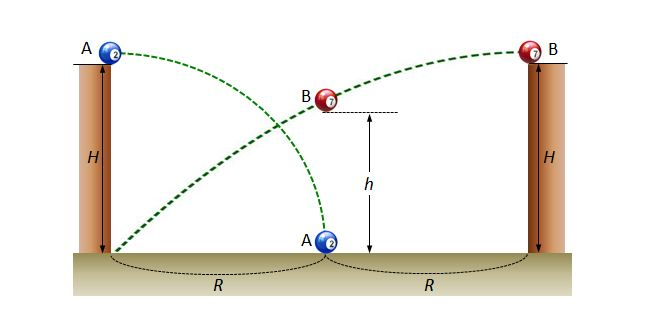# Projectile motion of ballsTwo balls $A$ and $B$ are thrown horizontally from a height of $H,$ as shown above. The horizontal throw lengths of $A$ and $B$ are $R$ and $2R,$ respectively. Let $h$ be the height of $B$ when its horizontal throw length is $R.$ What is the ratio $h:H?$

×